# Introduction

Can you define the notion of a volume of a shape? For example, what does ”volume” mean for a ball of radius 3? If you think about it, this is not a simple question. You probably understand intuitively what ”volume” and ”area” mean, but giving a proper definition might be tricky. The goal of this article is to provide you a view of a more formal mindset about defining volume and area, and to give you a brief introduction to measure theory.

# Back to school

To begin with, lets go way back to when you first learned about volume. In fact, let’s concentrate on area instead of volume right now for simplicity, since volume is essentially a higher-dimensional extension of area.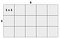Source: image created by the author.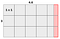Source: image created by the author.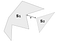The total area of a shape, made by ”gluing” together these two non-intersecting shapes of areas 𝑆₁ and 𝑆₂, is 𝑆₁ + 𝑆₂. Source: image created by the author.

# Starting to formalize our definition

Let’s focus on only defining volume, and saying that area is just a two-dimensional variant of volume, for the sake of simplicity.

1. Volume is additive, that is, for two non-intersecting sets 𝐴 and 𝐵, such that both of them are subsets of ℝ (so, for two non-intersecting sets of ”points”), the volume of their union 𝐴 ∪ 𝐵 is equal to the sum of the volume of 𝐴 and the volume of 𝐵.

# Rectangles and their Jordan measure

Let’s consider real-valued intervals of type [𝑎, 𝑏) = {all real 𝑥, such that 𝑎 ≤ 𝑥 < 𝑏} (it is important to note that we only consider intervals, where 𝑎 ≤ 𝑏, so let’s invalidate all other notation), more specifically, Cartesian products of these intervals, that is,

# Bounded sets, infimum, and supremum

Before we can proceed to defining the Jordan measure on arbitrary sets, we need to understand what a bounded set is, and also what ”infimum” and ”supremum” are. If you are already familiar with these topics, you can safely skip this part.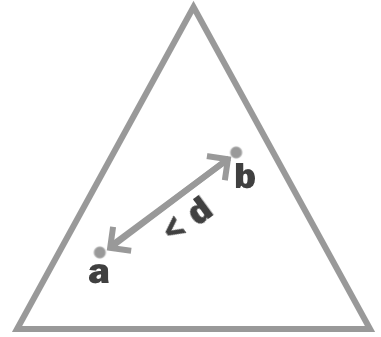This triangle (with real-valued coordinates) is a bounded set in ℝ², since there exists some real number 𝑑,such that the distance between any two points in the triangle is less than 𝑑. Source: image created by the author.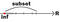In this example, 𝑆 = ℝ. Source: image created by the author.

# Jordan measure of abstract sets

Since we already formally defined volume for rectangles, let’s use them to ”approximate” the volume of all other shapes (and sets, for which it ”makes sense” to have volume defined for).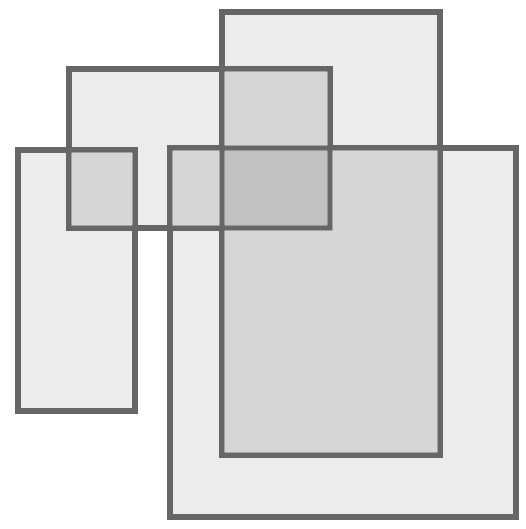Note: I didn’t draw the dashed sides like in the previous pictures, for the sake of simplicity, but,strictly speaking, they should be there. Source: image created by the author.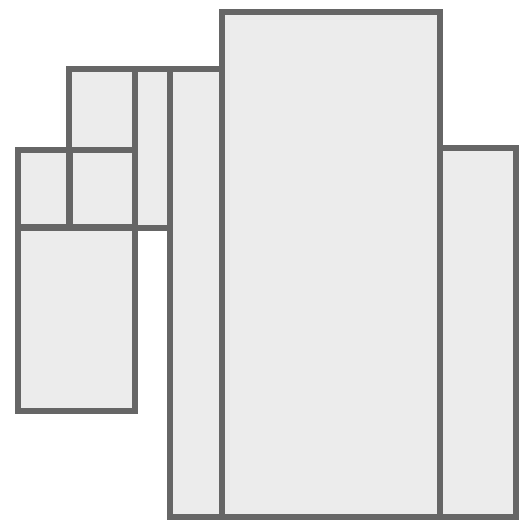Source: image created by the author.